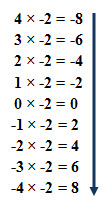### Ten Things That Require Zero Talent

I am an instructor at a community college. Many of my colleagues have unique  posters hanging outside their doors. I found this one by a co-worker's office who teaches English. I believe it is something we can all use during this difficult time when the coronavirus requires that most of us are to remain at home.

### Why does a Negative number times a Negative Number Equal a Positive Number?

Have you ever wondered why a negative number times a negative number equals a positive number? As my mathphobic daughter would say, "No, Mom. Math is something I never think about!" Well, for all of us who tend to be left brained people, the question can be answered by using a pattern. After all, all math is based on patterns!Let's examine 4 x -2 which means four sets of -2. Using the number line above, start at zero and move left by twos, four times. Voila! The answer is -8. Locate -8 on the number line above.

Now try 3 x -2. Again, begin at zero on the number line, but this time move left by twos, three times. Ta-dah! We arrive at -6. Therefore, 3 x -2 = -6.

On the left is what the mathematical sequence looks like. Moving down the sequence, observe that the farthest left hand column decreases by one each time, while the -2 remains constant. Simultaneously, the right hand answer column increases by 2 each time. Therefore, based on this mathematical pattern, we can conclude that a negative number times a negative number equals a positive number!!!!

Isn't Mathematics Amazing?